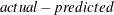# The MODEL Procedure

### Equation Translations

Equations written in normalized form are always automatically converted to general form equations. For example, when a normalized form equation such as

   y = a + b*x;


is encountered, it is translated into the equations

   PRED.y = a + b*x;
RESID.y = PRED.y - ACTUAL.y;
ERROR.y = PRED.y - y;


If the same system is expressed as the following general form equation, then this equation is used unchanged.

   EQ.y = y - (a + b*x);


This makes it easy to solve for arbitrary variables and to modify the error terms for autoregressive or moving average models.

Use the LIST option to see how this transformation is performed. For example, the following statements produce the listing shown in Figure 19.87.

proc model data=line list;
y = a1 + b1*x1 + c1*x2;
fit y;
run;


Figure 19.87: LIST Output

The MODEL Procedure

Listing of Compiled Program Code
Stmt Line:Col Statement as Parsed
1 4268:4 PRED.y = a1 + b1 * x1 + c1 * x2;
1 4268:4 RESID.y = PRED.y - ACTUAL.y;
1 4268:4 ERROR.y = PRED.y - y;

PRED.Y is the predicted value of Y, and ACTUAL.Y is the value of Y in the data set. The predicted value minus the actual value, RESID.Y, is then the error term,, for the original Y equation. Note that the residuals obtained from the OUTRESID option in the OUT=dataset for both the FIT and SOLVE statements are defined as, the negative of RESID.Y. See the section Syntax: MODEL Procedure for details. ACTUAL.Y and Y have the same value for parameter estimation. For solve tasks, ACTUAL.Y is still the value of Y in the data set but Y becomes the solved value; the value that satisfies PRED.Y – Y = 0.

The following are the equation variable definitions.

EQ.

The value of an EQ.-prefixed equation variable (normally used to define a general form equation) represents the failure of the equation to hold. When the EQ.name variable is 0, the name equation is satisfied.

RESID.

The RESID.name variables represent the stochastic parts of the equations and are used to define the objective function for the estimation process. A RESID.-prefixed equation variable is like an EQ.-prefixed variable but makes it possible to use or transform the stochastic part of the equation. The RESID. equation is used in place of the ERROR. equation for model solutions if it has been reassigned or used in the equation.

ERROR.

An ERROR.name variable is like an EQ.-prefixed variable, except that it is used only for model solution and does not affect parameter estimation.

PRED.

For a normalized form equation (specified by assignment to a model variable), the PRED.name equation variable holds the predicted value, where name is the name of both the model variable and the corresponding equation. (PRED.-prefixed variables are not created for general form equations.)

ACTUAL.

For a normalized form equation (specified by assignment to a model variable), the ACTUAL.name equation variable holds the value of the name model variable read from the input data set.

DERT.

The DERT.name variable defines a differential equation. Once defined, it might be used on the right-hand side of another equation.

H.

The H.name variable specifies the functional form for the variance of the named equation.

GMM_H.

This is created for H.vars and is the moment equation for the variance for GMM. This variable is used only for GMM.

   GMM_H.name = RESID.name**2 - H.name;

MSE.

The MSE.y variable contains the value of the mean squared error for y at each iteration. An MSE. variable is created for each dependent/endogenous variable in the model. These variables can be used to specify the missing lagged values in the estimation and simulation of GARCH type models.

  demret = intercept ;
h.demret = arch0 +
arch1 * xlag( resid.demret ** 2, mse.demret) +
garch1 * xlag(h.demret, mse.demret) ;

NRESID.

This is created for H.vars and is the normalized residual of the variable <name >. The formula is

   NRESID.name = RESID.name/ sqrt(H.name);


The three equation variable prefixes, RESID., ERROR., and EQ. allow for control over the objective function for the FIT, the SOLVE, or both the FIT and the SOLVE stages. For FIT tasks, PROC MODEL looks first for a RESID.name variable for each equation. If defined, the RESID.-prefixed equation variable is used to define the objective function for the parameter estimation process. Otherwise, PROC MODEL looks for an EQ.-prefixed variable for the equation and uses it instead.

For SOLVE tasks, PROC MODEL looks first for an ERROR.name variable for each equation. If defined, the ERROR.-prefixed equation variable is used for the solution process. Otherwise, PROC MODEL looks for an EQ.-prefixed variable for the equation and uses it instead. To solve the simultaneous equation system, PROC MODEL computes values of the solution variables (the model variables being solved for) that make all of the ERROR.name and EQ.name variables close to 0.